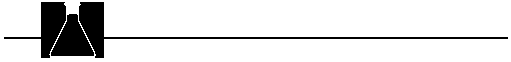## Tips For The Teachers

Language of Chemistry

1. The term "formula unit" is used in this module to refer to the smallest unit of a substance of a defined formula. For example, one formula unit of CO 3 2- refers to one CO32- ion. One formula unit of Ne would be one Ne atom. This terminology is especially useful when referring to ionic compounds because "molecules" of solid ionic compounds do not exist. One formula unit of NaCl, consists of one Na+ ion and one Cl- ion. Likewise, a formula unit of CuSO4* 5 H2O contains one Cu2+ ion, one SO42- ion, and 5 H 2O molecules. Because the molar mass of any substance is defined on the basis of its formula, the use of the term "formula unit" is not necessary. It is used here to avoid referring to atoms, molecules, ions, etc. each time we refer to moles.
2. Due to the SI definition of mole, there is no official basis for using the terms "gram moles," "pound moles," "ton moles,"etc. even though these are sometimes still encountered. The molar mass is the mass of one mole of any species expressed in grams.
3. "Formula weight," "gram formula weight," "molecular weight," "gram molecular weight," and "atomic weight" are terms that refer to moles of specific categories of substances. These are used synonymously with "molar mass," which remains the preferred term.
4. The meaning of formulas in terms of moles (rather than atoms) should be stressed so students begin to think "moles" rather than "atoms," "molecules" or "formula units" when they see a formula or interpret its meaning. This will greatly facilitate calculations encountered later in this module (percent composition and empirical formula) and stoichiometry problems, which follow soon after this topic in most texts.
5. The empirical formula is the simplest formula for a substance. In the empirical formula the subscripts are reduced to the smallest possible integers. The formulas for many ionic substances are empirical formulas; however, molecules of covalent substances are frequently a multiple of the empirical formula. The formula for a molecule is called the molecular formula. The empirical formula for a covalent compound is found by dividing all the subscripts by the largest common factor (e.g., the empirical formula for C6H12O6 is CH2 O).

Common Student Misconceptions

1. "Equal volumes or equal masses of different substances contain the same number of moles (or atoms/molecules/formula units)."
It is true that equal volumes of gases contain the same number of moles of gas molecules (assuming equal temperatures and pressures), but such an assertion is wide of the mark for liquids or solids. No such generalizations are possible for the masses of substances-whether in gaseous state or not.

2. "The term `mole' refers only to `molecules'. "
This is particularly problematic if the mole concept is only applied to molecules (or formula units). It is advisable to apply the concept of moles also to ions, electrons, and/or other entities so that students will not associate "mole" exclusively with the term "molecule."

3. "One mole of various substances may contain different numbers of units of those substances."
Although Avogadro's number (6.02 x 1023 ) is such a large number that it cannot be meaningfully visualized, it is important that students conceive of one mole of a substance as containing the same number of units as one mole of any other substance. However, you must work hard to destroy the idea that equal masses or equal volumes of two substances contain the same number of moles, as was highlighted in Misconception 1.